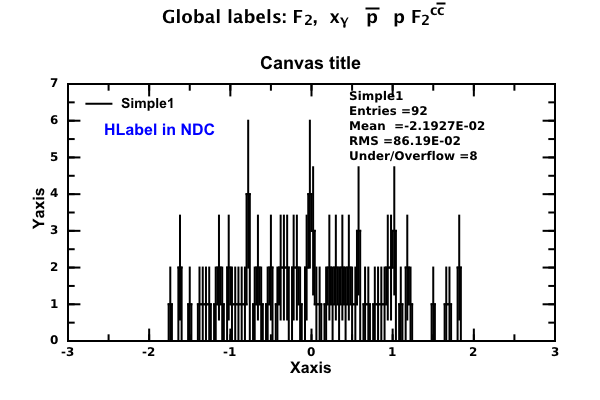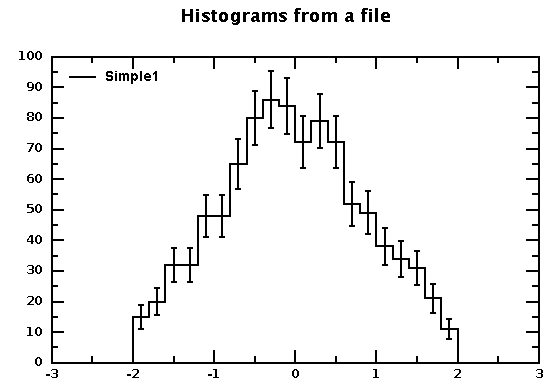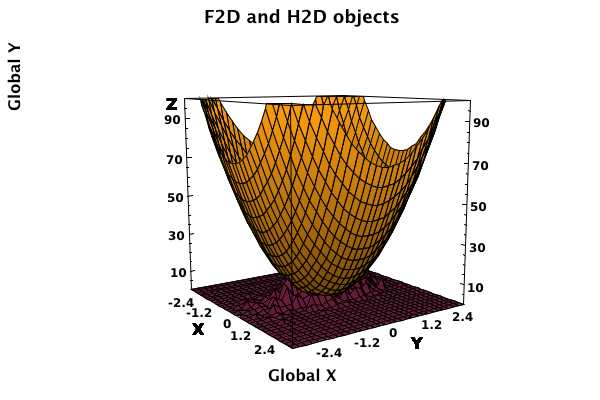# DMelt:DataAnalysis/3 Histograms

Limitted access. First login to DataMelt if you are a full DataMelt member. Then login to HandWiki as a user.

# Histograms

A graphical representation of the distribution can be done using histograms. Read the Histogram article. To construct a histogram representing a density distribution of some variable one should follow these 2 steps: construct a histogram object using thejhplot.H1D class and then fill it.

Here is an simple example of how to build a histogram with 100 bins between 0 and 5:

from jhplot import *
h1=H1D("Test histogram",100,0,5)

Use the method "fill" to fill this histogram.

## Plotting a histogram

This is an example using the Jython code and the jhplot package (it is rather trivial to rewrite it in Java):

from java.awt import Color
from java.util import Random
from jhplot  import HPlot,H1D,HTable,HLabel

'''
This is a multiline comment with a LaTeX equation
$$z=x*\alpha *\int^{100}_{k}$$
'''

c1 = HPlot("Canvas",600,400,1, 1)
# c1.doc() # view documetation
c1.setGTitle("Global labels: F_{2},  x_{&gamma;}  #bar{p}p F_{2}^{c#bar{c}}"); #put title
c1.visible(1)
c1.setAutoRange()
h1 = H1D("Simple1",100, -2, 2.0)
rand = Random()
# fill histogram
for i in range(100):
h1.fill(rand.nextGaussian())

c1.draw(h1)

c1.setAutoRange()
h1.setPenWidthErr(2)
c1.setNameX("Xaxis")
c1.setNameY("Yaxis")
c1.setName("Canvas title")
c1.drawStatBox(h1)

# make exact copy
# h2=h1.copy()
# show as a table
# HTable(h1)
# c1.draw(h2)

# print statistics
stat=h1.getStat()
for key in  stat:
print key , '\t', stat[key]

# set HLabel in the normilised coordinate system
lab=HLabel("HLabel in NDC", 0.15, 0.7, "NDC")
lab.setColor(Color.blue)

c1.update()

# export to some image (png,eps,pdf,jpeg...)
# c1.export(Editor.DocMasterName()+".png");
# edit the image
# IEditor(Editor.DocMasterName()+".png");

The output with a statistical summary is plotted as well (the method drawStatBox()). By default, the plot shows statistical uncertainties in each bin.In the above example, "100" is the number of bins between -2 and 2, thus all bins are of the same size. You can get some information about histograms as:

• getBinSize() Get bin width in case of fixed-size bins
• getDensity() Get a density distribution dividing each bin of the histogram by the bin width and the total number of heights for all bins.
• getProbability() Return a probability distribution derived from a histogram.
• integral(i1,i2) Integrate a histogram between two bin indices

You can get a complete different look and feel using various attributes of thejhplot.H1D orjhplot.HPlot class.

You can use different canvases also (see Section Canvases. For example, replacingjhplot.HPlot withjhplot.HPlotter leads to the image shown below:

## Statistics

You can get detailed statistics on a given histogram using the method getStat(). It returns a map (for JAVA) or Python dictionary (for Jython) where each statistical characteristics can be accessed using a key, such as mean, RMS, variance, error on the mean at.

stat=h1.getStat()  # get PYTHON dictionary with statistics
for key in  stat:
print key , 't', stat[key]

This will print the following values:

overflowBin 	     4.0
error 	             0.0824244396904
underflowBin 	     4.0
rms 	             0.793596244181
variance             0.625028519761
allEntries 	     100.0
maxBinHeight 	      5.0
minBinHeight 	      0.0
mean                  -0.0690396916107
entries 	      92.0
underflowHeight       4.0
stddev 	              0.790587452317
overflowHeight 	      4.0

## Histogram input/output

As any object in DataMelt, you can serialize histogram into a file and then read it back. Here we show a simple example how to write a histogram into a human-readable text file (jdat) and then read it back.

This example also shows a canvas with the histogram from a file. Open the output.jdat file and study. Most tags are optional. The histogram entries are stored between "data" tag. The expected output after reading this histogram from the file is shown here:## Histogram conversions

Histograms can be generated from F1D and F2D functions as explained in the Functions section. The opposite is not true. Histograms can be converted to the P1D or P2D data points as explained in Data structures section.

# Histograms in 2D

Build a histogram in 2 dimensions using the Java classjhplot.H2D class. This is an example using the JHPLOT package (here we are using again Jython syntax, instead of Java):

The output with statistical summary is shown here. By default, the plot shows statistical uncertainties in each bin.# Histograms in 3D

Similarly, histograms can be defined in 3D using thejhplot.H3D class.

# Histograms with variable bin size

One can use also variable-size bins as:

h1 = H1D("Variable-size bins",[-2,-1,0,2,10])

where the list used in the H1D constructor specifies edges of the bins. Similarly, one can define H2D and H3D histogram by passing 2 lists (one for X, one for Y) or 3 lists (X,Y,Z).

# Histogram operations

The histogram classes support many mathematical operations (division, subtraction, multiplication, scaling, shifting, smoothing etc). Histogram arithmetic can be done with the method "oper(h,"New Title","operation")", where "h" is an object represented a histogram which is used to subtract, divide, multiply and add. All these operations should be defined by a string operation as "-, /, *, +", and the histograms must have the same binning. It should also be noted that all such operations take into account propagation of statistical errors for each bin assuming that histograms do not correlate.

from java.util import Random
from jhplot  import *
h1 = H1D("First",10, -2.0, 2.0)
h2 = H1D("Second",10, -2.0, 2.0)
r = Random()
for i in range(5000):  h1.fill(r.nextGaussian())
for i in range(5000):  h2.fill(r.nextGaussian())
h3=h1.oper(h2,"subtract","-")
h6=h1.oper(h2,"divide","/")